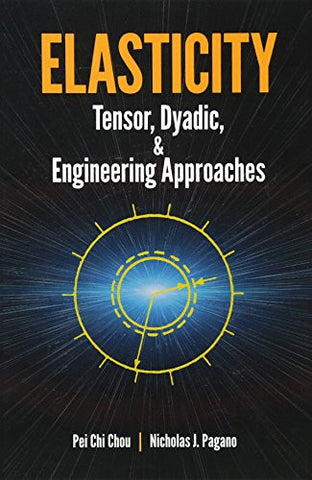# Elasticity: Tensor, Dyadic, and Engineering Approaches (Dover Civil and Mechanical Engineering)

• \$24.34
• Save \$13
• Binding: Paperback
• Author: Pei Chi Chou;Nicholas J. Pagano
• Publish Date: 1992-01-17

Attention : For textbook, access codes and supplements are not guaranteed with used items.

Written for advanced undergraduates and beginning graduate students, this exceptionally clear text treats both the engineering and mathematical aspects of elasticity. It is especially useful because it offers the theory of linear elasticity from three standpoints: engineering, Cartesian tensor, and vector-dyadic. In this way the student receives a more complete picture and a more thorough understanding of engineering elasticity. Prerequisites are a working knowledge of statics and strength of materials plus calculus and vector analysis.
The first part of the book treats the theory of elasticity by the most elementary approach, emphasizing physical significance and using engineering notations. It gives engineering students a clear, basic understanding of linear elasticity. The latter part of the text, after Cartesian tensor and dyadic notations are introduced, gives a more general treatment of elasticity. Most of the equations of the earlier chapters are repeated in Cartesian tensor notation and again in vector-dyadic notation. By having access to this threefold approach in one book, beginning students will benefit from cross-referencing, which makes the learning process easier.
Another helpful feature of this text is the charts and tables showing the logical relationships among the equations â especially useful in elasticity, where the mathematical chain from definition and concept to application is often long. Understanding of the theory is further reinforced by extensive problems at the end of each chapter.